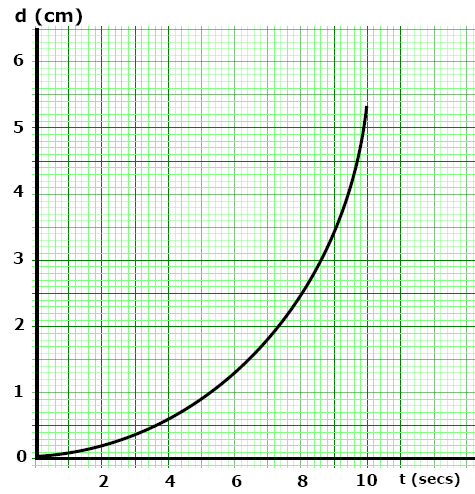Try this at home!

School blocks YouTube? Use the file below

| Onsite |

Review

Video Instruction

showmethephysics.com

II. Motion Diagrams - plots of motion data

Detailed, visual way
of describing motion

Both plots show an increasing displacement

Describe the velocity for each.Constant Velocity Increasing Velocity

A. Displacement vs. Time Plot

Ex)

(d) Displacement (m)Calculate the Velocity
from 2 to 4 sec

V = ∆d/∆t

= (10. m - 5.0 m)/(4.0 s - 2.0 s)

= +2.5 m/s

(d) Displacement (m)Velocity from 4 to  6 seconds?

= +2.5 m/s

(d) Displacement (m)What is happening from 6 to 8 seconds?

Motion Map

5 m in 2 sec / 5 m in 2sec / 5 m in 2 sec10 m backward in 2 sec

(d) Displacement (m)Slope of a d vs. t graph  = velocity

Describe the various motions shown in this plotA to B?

Constant VelocityB to C?

Increasing V,
then Decreasing VD?

Rest

V = 0D to E ?

Increasing VMotion Diagrams II